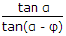# Online Mechanical Engineering Test - Theory of Machines Test 1Loading Test...

Instruction:

• This is a FREE online test. DO NOT pay money to anyone to attend this test.
• Total number of questions : 20.
• Time alloted : 30 minutes.
• Each question carry 1 mark, no negative marks.
• DO NOT refresh the page.
• All the best :-).

1.

The partial balancing of reciprocating parts in locomotives produces

A.
 hammer blowB.
 swaying coupleC.
 variation in tractive force along the line of strokeD.
 all of the above2.

Maximum fluctuation of energy in a flywheel is equal to (where I = Mass moment of inertia of the flywheel, E = Maximum fluctuation of energy, CS = Coefficient of fluctuation of speed, and ω = Mean angular speed =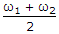)

A.
 Iω(ω1 - ω2)B.
 Iω2CSC.
 2ECSD.
 all of these3.

If the controlling force line for a spring controlled governor when produced intersects the Y-axis at the origin, then the governor is said to be

A.
 stableB.
 unstableC.
 isochronousD.
 none of these4.

When the crank is at the outer dead centre, in a reciprocating steam engine, then the acceleration of the piston will be

A.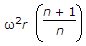B.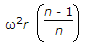C.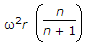D.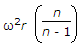5.

In vibration isolation system, if ω/ωn > 2, then for all values of damping factor, the trans-missibility (i.e. the ratio of the force transmitted to the force applied) will be less than unity.

A.
 TrueB.
 False6.

A Hartnell governor is a

A.
 dead weight governorB.
 pendulum type governorC.
 spring loaded governorD.
 inertia governor7.

The minimum number of teeth on the pinion which will mesh with any gear without interference for 20° full depth involute teeth will be

A.
 12B.
 14C.
 18D.
 248.

The coriolis component of acceleration leads the sliding velocity by

A.
 45°B.
 90°C.
 135°D.
 180°9.

The length of a simple pendulum which gives the same frequency as the compound pendulum, is

A.
 kG + l1B.
 kG2 + l1C.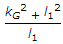D.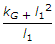10.

A slender shaft supported on two bearings at its ends carries a disc with an eccentricity e from the axis of rotation. The critical speed of the shaft is Nc. If the disc is replaced by a second one of same mass but mounted with an eccentricily 2 e, then the critical speed of the shaft in the second case is

A.
 Nc/2B.
 Nc/2C.
 NcD.
 2 Nc11.

The unbalanced force due to revolving masses

A.
 varies in magnitude but constant in directionB.
 varies in direction but constant in magnitudeC.
 varies in magnitude and direction bothD.
 constant in magnitude and direction both12.

The Grubler's criterion for determining the degrees of freedom (n) of a mechanism having plane motion is (where l = Number of links, and j = Number of binary joints)

A.
 n = (l - 1) - jB.
 n = 2(l - 1) - 2jC.
 n = 3(l - 1) - 2jD.
 n = 4(l - 1) - 3j13.

The angle of inclination of the plane, at which the body begins to move down the plane is called

A.
 angle of frictionB.
 angle of reposeC.
 angle of projectionD.
 none of these14.

When the load on the engine increases, it becomes necessary to increase the supply of working fluid and when the load decreases, less working fluid is required. The supply of the working fluid to the engine is controlled by a

A.
 D-slide valveB.
 governorC.
 Meyer's expansion valveD.
 flywheel15.

The Klein's method of construction for reciprocating engine mechanism

A.
 is a simplified version of instantaneous centre methodB.
 utilises a quadrilateral similar to the diagram of mechanism for reciprocating engineC.
 enables determination of coriolis componentD.
 is based on the acceleration diagram16.

Power of a governor is the

A.
 mean force exerted at the sleeve for a given percentage change of speedB.
 workdone at the sleeve for maximum equilibrium speedC.
 mean force exerted at the sleeve for maximum equilibrium speedD.
 none of the above17.

Under logarithmic decrement, the amplitude of successive vibrations are

A.
 constantB.
 in arithmetic progressionC.
 in geometric progressionD.
 in logarithmic progression18.

The centre of suspension and centre of percussion are not interchangeable.

A.
 CorrectB.
 Incorrect19.

The primary unbalanced force of reciprocating masses is inversely proportional to the crank radius.

A.
 YesB.
 No20.

Efficiency of a screw jack is given by

A.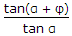B.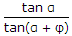C.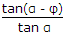D.